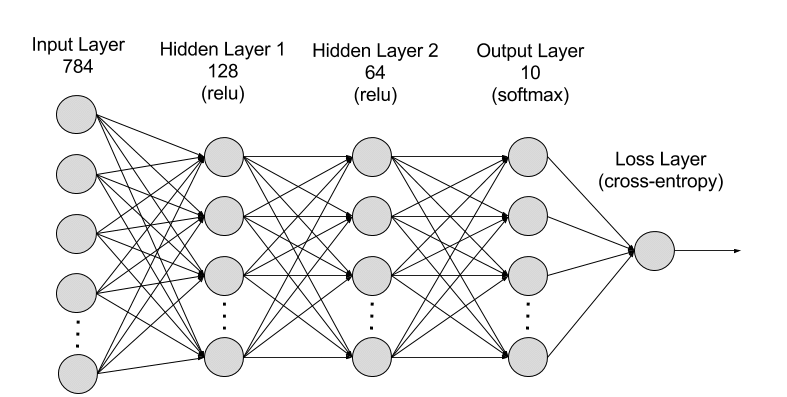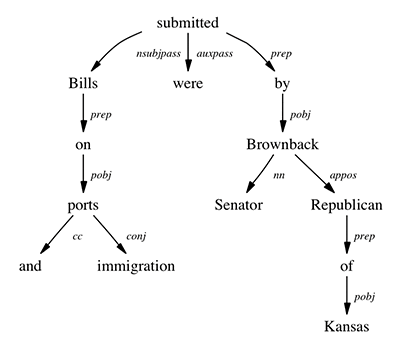# 介绍 Gluon 这一易于使用的灵活深度学习编程接口

## (1) 简单、容易理解的代码

``````# 第一步是初始化您的模型
net = gluon.nn.Sequential()
# 接下来，定义您的模型架构
with net.name_scope():
net.add(gluon.nn.Dense(128, activation="relu")) # 第一层 - 128 个节点
net.add(gluon.nn.Dense(64, activation="relu")) # 第二层 - 64 个节点## (2) 灵活的结构

Gluon 方法则另辟蹊径。它将训练算法和神经网络模型组合在一起，在开发过程中提供了灵活性，而不会牺牲性能。这种方法的核心是 Gluon trainer 方法，该方法用于对模型进行训练。 trainer 方法依托于 MXNet autograd 库，该库用于自动计算导数 (即梯度)。导数 是一种数学运算，用于测量变量的变化率。这是训练算法的必要输入。 autograd 库可以有效地实施这些数学运算，并且对于支持 Gluon 提供的灵活性至关重要。现在，您可以定义由一个简单的嵌套 for 循环构成的训练算法，只需结合使用 autogradtrainer即可。

``````epochs = 10

for e in range(epochs):
for i, batch in enumerate(train_data):
data = batch.data
label = batch.label
output = net(data) # 前向迭代
loss = softmax_cross_entropy(output, label)
loss.backward()
trainer.step(data.shape)``````

## (3) 动态图表

• “Michael threw the football in front of the player.”
• “The ball was thrown short of the target by Michael.”(斯坦福自然语言处理组)

``````def forward(self, F, inputs, tree):
children_outputs = [self.forward(F, inputs, child)
for child in tree.children]
#在模型定义和训练过程中，基于每个
#输入句子的语法结构来构建神经网络
…
``````

## (4) 高性能

``````net = nn.HybridSequential()
with net.name_scope():
net.add(nn.Dense(128, activation="relu")) # 第一层 - 128 个节点
net.add(nn.Dense(64, activation="relu")) # 第二层 - 64 个节点
``````

``net.hybridize()``

## Gluon 入门

``````import mxnet as mx
from mxnet import gluon, autograd, ndarray``````

``````# 使用本机 MXNet utils 函数导入 MNIST
data = mx.test_utils.get_mnist()

# 设置训练数据并重新整理图片
train_data = data['train_data'].reshape((-1, 784))
train_label = data['train_label']

# 设置测试数据并重新整理图片
test_data = data['test_data'].reshape((-1, 784))
test_label = data['test_label']

# 将数据转换为 NDArray
train_data_mx = mx.nd.array(train_data)
train_label_mx = mx.nd.array(train_label)
test_data_mx = mx.nd.array(test_data)
test_label_mx = mx.nd.array(test_label)``````

``````batch_size = 32
train_data = mx.io.NDArrayIter(train_data_mx, train_label_mx, batch_size,
shuffle=True)           ``````

``````# 第一步是初始化您的模型
net = gluon.nn.Sequential()
# 接下来，定义您的模型架构
with net.name_scope():
net.add(gluon.nn.Dense(128, activation="relu")) # 第一层 - 128 个节点
net.add(gluon.nn.Dense(64, activation="relu")) # 第二层 - 64 个节点

``````# 我们从正态分布中所有模型参数的
# 随机值开始，标准偏差为 0.05
net.collect_params().initialize(mx.init.Normal(sigma=0.05))

# 我们选择使用 softmax 交叉熵损耗函数
# 来测量模型能够预测正确答案的程度
softmax_cross_entropy = gluon.loss.SoftmaxCrossEntropyLoss()

# 我们选择使用随机梯度下降 (sgd) 训练算法，
# 并将学习速率超参数设置为 .1
trainer = gluon.Trainer(net.collect_params(), 'sgd', {'learning_rate': .1})``````

``````epochs = 10

for e in range(epochs):
for i, batch in enumerate(train_data):
data = batch.data
label = batch.label
output = net(data) # 前向迭代
loss = softmax_cross_entropy(output, label)
loss.backward()
trainer.step(data.shape)``````

``````acc = mx.metric.Accuracy()# 初始化准确度指标
output = net(test_data_mx) # 通过神经网络运行测试数据
predictions = ndarray.argmax(output, axis=1) # 测试数据的预测
acc.update(preds=predictions, labels=test_label_mx) # 计算准确度
print(acc)
``````

### 作者简介Vikram Madan 是 AWS 深度学习高级产品经理。他负责开发让深度学习引擎变得更易于使用的产品，工作重点是开源 Apache MXNet 引擎。在业余时间，他喜欢长跑以及看纪录片。

TAGS: